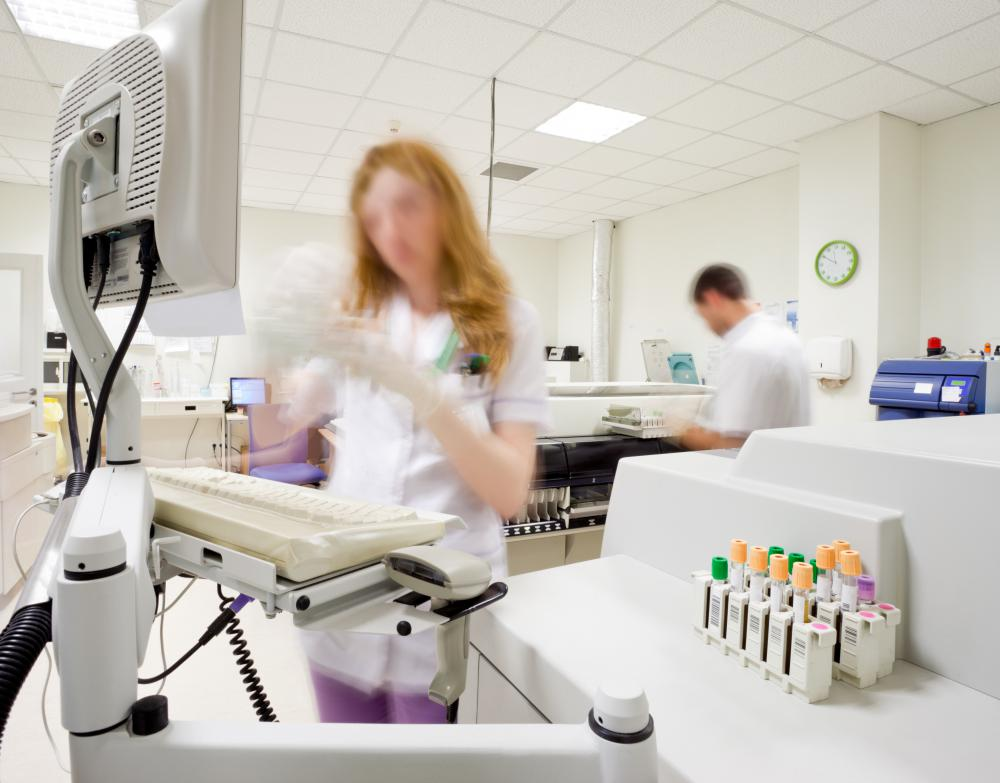# What is Hypothesis Testing?

Daniel Liden
Daniel LidenHypothesis testing often involves mathematical formulas.

Hypothesis testing is a common practice in science that involves conducting tests and experiments to see if a proposed explanation for an observed phenomenon works in practice. A hypothesis is a tentative explanation for some kind of observed phenomenon, and is an important part of the scientific method. The scientific method is a set of steps that is commonly employed by those in scientific fields to give scientific explanations for various phenomena.Medical research often tests hypotheses with large quantities of data.

Any tentative explanation can be referred to as a hypothesis if it can be submitted to hypothesis testing. There are, however, a set of guidelines for an explanation to be considered a true scientific hypothesis. The first major point is testability; a scientific hypothesis must be able to proceed to the stage of hypothesis testing to be considered a scientifically legitimate hypothesis. It is generally suggested that a hypothesis be relatively simple, though this is not always possible. Hypotheses must also be able to explain the phenomena under any set of conditions; if a hypothesis can only explain a phenomenon in one set of conditions, it is generally considered unacceptable.

Hypotheses are generally considered useful only if they are likely to improve on the current body of knowledge on a subject and pave the way for greater knowledge to be acquired in the future. Also, a hypothesis is generally not acknowledged if it defies other commonly recognized knowledge. If a hypothesis meets all of these requirements, it will typically proceed to the hypothesis testing phase.

In hypothesis testing, the testers seek to discover evidence that either validates or disproves a given hypothesis. Usually, this involves a series of experiments being conducted in many different conditions. If the hypothesis does not stand up to the tests in all conditions, something is usually wrong with the hypothesis and a new one must be formed to take the new information into account. The new hypothesis is submitted to the same hypothesis testing. If it passes and is not proven wrong, it can eventually be considered a scientific theory or law, though nothing in science can be proven to be absolutely true.

One common method of hypothesis testing is known as statistical hypothesis testing, and typically deals with large quantities of data. Experiments and tests are conducted and the data is collected. If the data collected shows that it is unlikely that the results occurred by chance, it is considered statistically significant and can be used to support a hypothesis.

## You might also Like## Discussion Comments

FernValley

It is also common for multiple teams of scientists to test a hypothesis using the same experiments before they accept a hypothesis as proven.

sherlock87

Hypothesis testing is one of the few things I remember from the science classes I took in high school. Specifically, the idea that nothing in science is entirely true, it is just true until a new hypothesis is proven that rules it out, or if the original hypothesis is dis-proven somehow. That has always stuck with me, because I feel like most people are way too trusting of "science", or at least the science they read about in magazines or see on television shows.

Forgot password?
###### Register:
•Hypothesis testing often involves mathematical formulas.
•Medical research often tests hypotheses with large quantities of data.